Electrolytic Cells

Electrolytic Cells

 The Electrolysis of Molten NaCl The Electrolysis of Aqueous NaCl The Electrolysis of Water Faraday's Law

Voltaic cells use a spontaneous chemical reaction to drive an electric current through an external circuit. These cells are important because they are the basis for the batteries that fuel modern society. But they aren't the only kind of electrochemical cell. It is also possible to construct a cell that does work on a chemical system by driving an electric current through the system. These cells are called electrolytic cells. Electrolysis is used to drive an oxidation-reduction reaction in a direction in which it does not occur spontaneously.

The Electrolysis of Molten NaCl

An idealized cell for the electrolysis of sodium chloride is shown in the figure below. A source of direct current is connected to a pair of inert electrodes immersed in molten sodium chloride. Because the salt has been heated until it melts, the Na+ ions flow toward the negative electrode and the Cl- ions flow toward the positive electrode.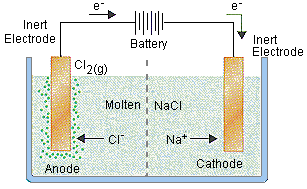When Na+ ions collide with the negative electrode, the battery carries a large enough potential to force these ions to pick up electrons to form sodium metal.

 Negative electrode (cathode): Na+ + e-Na

Cl- ions that collide with the positive electrode are oxidized to Cl2 gas, which bubbles off at this electrode.

 Positive electrode (anode): 2 Cl-Cl2 + 2 e-

The net effect of passing an electric current through the molten salt in this cell is to decompose sodium chloride into its elements, sodium metal and chlorine gas.

 Electrolysis of NaCl: Cathode (-): Na+ + e-Na Anode (+): 2 Cl-Cl2 + 2 e-

The potential required to oxidize Cl- ions to Cl2 is -1.36 volts and the potential needed to reduce Na+ ions to sodium metal is -2.71 volts. The battery used to drive this reaction must therefore have a potential of at least 4.07 volts.

This example explains why the process is called electrolysis. The suffix -lysis comes from the Greek stem meaning to loosen or split up. Electrolysis literally uses an electric current to split a compound into its elements.

 electrolysis 2 NaCl(l)2 Na(l) + Cl2(g)

This example also illustrates the difference between voltaic cells and electrolytic cells. Voltaic cells use the energy given off in a spontaneous reaction to do electrical work. Electrolytic cells use electrical work as source of energy to drive the reaction in the opposite direction.

The dotted vertical line in the center of the above figure represents a diaphragm that keeps the Cl2 gas produced at the anode from coming into contact with the sodium metal generated at the cathode. The function of this diaphragm can be understood by turning to a more realistic drawing of the commercial Downs cell used to electrolyze sodium chloride shown in the figure below.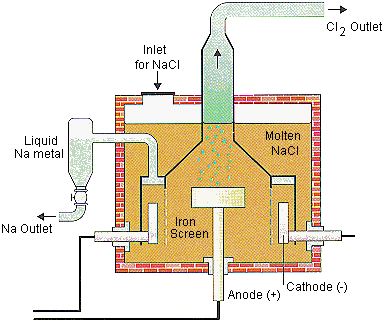Chlorine gas that forms on the graphite anode inserted into the bottom of this cell bubbles through the molten sodium chloride into a funnel at the top of the cell. Sodium metal that forms at the cathode floats up through the molten sodium chloride into a sodium-collecting ring, from which it is periodically drained. The diaphragm that separates the two electrodes is a screen of iron gauze, which prevents the explosive reaction that would occur if the products of the electrolysis reaction came in contact.

The feed-stock for the Downs cell is a 3:2 mixture by mass of CaCl2 and NaCl. This mixture is used because it has a melting point of 580oC, whereas pure sodium chloride has to be heated to more than 800oC before it melts.The Electrolysis of Aqueous NaCl

The figure below shows an idealized drawing of a cell in which an aqueous solution of sodium chloride is electrolyzed.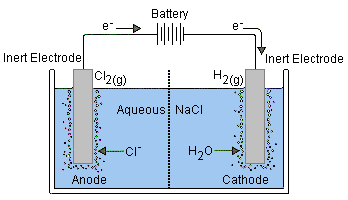Once again, the Na+ ions migrate toward the negative electrode and the Cl- ions migrate toward the positive electrode. But, now there are two substances that can be reduced at the cathode: Na+ ions and water molecules.

 Cathode (-): Na+ + e-Na Eored = -2.71 V 2 H2O + 2 e-H2 + 2 OH- Eored = -0.83 V

Because it is much easier to reduce water than Na+ ions, the only product formed at the cathode is hydrogen gas.

 Cathode (-): 2 H2O(l) + 2 e-H2(g) + 2 OH-(aq)

There are also two substances that can be oxidized at the anode: Cl- ions and water molecules.

 Anode (+): 2 Cl-Cl2 + 2 e- Eoox = -1.36 V 2 H2OO2 + 4 H+ + 4 e- Eoox = -1.23 V

The standard-state potentials for these half-reactions are so close to each other that we might expect to see a mixture of Cl2 and O2 gas collect at the anode. In practice, the only product of this reaction is Cl2.

 Anode (+): 2 Cl-Cl2 + 2 e-

At first glance, it would seem easier to oxidize water (Eoox = -1.23 volts) than Cl- ions (Eoox = -1.36 volts). It is worth noting, however, that the cell is never allowed to reach standard-state conditions. The solution is typically 25% NaCl by mass, which significantly decreases the potential required to oxidize the Cl- ion. The pH of the cell is also kept very high, which decreases the oxidation potential for water. The deciding factor is a phenomenon known as overvoltage, which is the extra voltage that must be applied to a reaction to get it to occur at the rate at which it would occur in an ideal system.

Under ideal conditions, a potential of 1.23 volts is large enough to oxidize water to O2 gas. Under real conditions, however, it can take a much larger voltage to initiate this reaction. (The overvoltage for the oxidation of water can be as large as 1 volt.) By carefully choosing the electrode to maximize the overvoltage for the oxidation of water and then carefully controlling the potential at which the cell operates, we can ensure that only chlorine is produced in this reaction.

In summary, electrolysis of aqueous solutions of sodium chloride doesn't give the same products as electrolysis of molten sodium chloride. Electrolysis of molten NaCl decomposes this compound into its elements.

 electrolysis 2 NaCl(l)2 Na(l) + Cl2(g)

Electrolysis of aqueous NaCl solutions gives a mixture of hydrogen and chlorine gas and an aqueous sodium hydroxide solution.

 electrolysis 2 NaCl(aq) + 2 H2O(l)2 Na+(aq) + 2 OH-(aq) + H2(g) + Cl2(g)

Because the demand for chlorine is much larger than the demand for sodium, electrolysis of aqueous sodium chloride is a more important process commercially. Electrolysis of an aqueous NaCl solution has two other advantages. It produces H2 gas at the cathode, which can be collected and sold. It also produces NaOH, which can be drained from the bottom of the electrolytic cell and sold.The dotted vertical line in the above figure represents a diaphragm that prevents the Cl2 produced at the anode in this cell from coming into contact with the NaOH that accumulates at the cathode. When this diaphragm is removed from the cell, the products of the electrolysis of aqueous sodium chloride react to form sodium hypo-chlorite, which is the first step in the preparation of hypochlorite bleaches, such as Chlorox.

Cl2(g) + 2 OH-(aq)Cl-(aq) + OCl-(aq) + H2O(l)Electrolysis of Water

A standard apparatus for the electrolysis of water is shown in the figure below.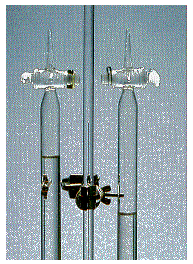electrolysis 2 H2O(l)2 H2(g) + O2(g)

A pair of inert electrodes are sealed in opposite ends of a container designed to collect the H2 and O2 gas given off in this reaction. The electrodes are then connected to a battery or another source of electric current.

By itself, water is a very poor conductor of electricity. We therefore add an electrolyte to water to provide ions that can flow through the solution, thereby completing the electric circuit. The electrolyte must be soluble in water. It should also be relatively inexpensive. Most importantly, it must contain ions that are harder to oxidize or reduce than water.

 2 H2O + 2 e-H2 + 2 OH- Eored = -0.83 V 2 H2OO2 + 4 H+ + 4 e- Eoox = -1.23 V

The following cations are harder to reduce than water: Li+, Rb+, K+, Cs+, Ba2+, Sr2+, Ca2+, Na+, and Mg2+. Two of these cations are more likely candidates than the others because they form inexpensive, soluble salts: Na+ and K+.

The SO42- ion might be the best anion to use because it is the most difficult anion to oxidize. The potential for oxidation of this ion to the peroxydisulfate ion is -2.05 volts.

 2 SO42-S2O82- + 2 e- Eoox = -2.05 V

When an aqueous solution of either Na2SO4 or K2SO4 is electrolyzed in the apparatus shown in the above figure, H2 gas collects at one electrode and O2 gas collects at the other.

What would happen if we added an indicator such as bromothymol blue to this apparatus? Bromothymol blue turns yellow in acidic solutions (pH < 6) and blue in basic solutions (pH > 7.6). According to the equations for the two half-reactions, the indicator should turn yellow at the anode and blue at the cathode.

 Cathode (-): 2 H2O + 2 e-H2 + 2 OH- Anode (+): 2 H2OO2 + 4 H+ + 4 e-Faraday's law of electrolysis can be stated as follows. The amount of a substance consumed or produced at one of the electrodes in an electrolytic cell is directly proportional to the amount of electricity that passes through the cell.

In order to use Faraday's law we need to recognize the relationship between current, time, and the amount of electric charge that flows through a circuit. By definition, one coulomb of charge is transferred when a 1-amp current flows for 1 second.

1 C = 1 amp-s

Example: To illustrate how Faraday's law can be used, let's calculate the number of grams of sodium metal that will form at the cathode when a 10.0-amp current is passed through molten sodium chloride for a period of 4.00 hours.

We start by calculating the amount of electric charge that flows through the cell.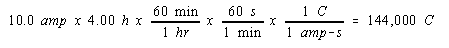Before we can use this information, we need a bridge between this macroscopic quantity and the phenomenon that occurs on the atomic scale. This bridge is represented by Faraday's constant, which describes the number of coulombs of charge carried by a mole of electrons.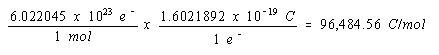Thus, the number of moles of electrons transferred when 144,000 coulombs of electric charge flow through the cell can be calculated as follows.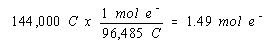According to the balanced equation for the reaction that occurs at the cathode of this cell, we get one mole of sodium for every mole of electrons.

 Cathode (-): Na+ + e-Na

Thus, we get 1.49 moles, or 34.3 grams, of sodium in 4.00 hours.The consequences of this calculation are interesting. We would have to run this electrolysis for more than two days to prepare a pound of sodium.

 Practice Problem 13:Calculate the volume of H2 gas at 25oC and 1.00 atm that will collect at the cathode when an aqueous solution of Na2SO4 is electrolyzed for 2.00 hours with a 10.0-amp current. Click here to check your answer to Practice Problem 13 Click here to see a solution to Practice Problem 13

We can extend the general pattern outlined in this section to answer questions that might seem impossible at first glance.

 Practice Problem 14:Determine the oxidation number of the chromium in an unknown salt if electrolysis of a molten sample of this salt for 1.50 hours with a 10.0-amp current deposits 9.71 grams of chromium metal at the cathode. Click here to check your answer to Practice Problem 14 Click here to see a solution to Practice Problem 14